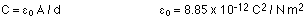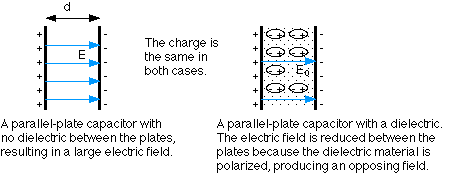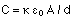## Capacitors and batteries

7-9-99

### Capacitors: devices for storing charge

A capacitor is a device for storing charge. It is usually made up of two plates separated by a thin insulating material known as the dielectric. One plate of the capacitor is positively charged, while the other has negative charge.

The charge stored in a capacitor is proportional to the potential difference between the two plates. For a capacitor with charge Q on the positive plate and -Q on the negative plate, the charge is proportional to the potential:

If C is the capacitance, Q = CV

The capacitance is a measure of the amount of charge a capacitor can store; this is determined by the capacitor geometry and by the kind of dielectric between the plates. For a parallel plate capacitor made up of two plates of area A and separated by a distance d, with no dielectric material, the capacitance is given by :Note that capacitance has units of farads (F). A 1 F capacitor is exceptionally large; typical capacitors have capacitances in the pF - microfarad range.

Dielectrics, insulating materials placed between the plates of a capacitor, cause the electric field inside the capacitor to be reduced for the same amount of charge on the plates. This is because the molecules of the dielectric material get polarized in the field, and they align themselves in a way that sets up another field inside the dielectric opposite to the field from the capacitor plates. The dielectric constant is the ratio of the electric field without the dielectric to the field with the dielectric:Note that for a set of parallel plates, the electric field between the plates is related to the potential difference by the equation:

for a parallel-plate capacitor: E = V / d

For a given potential difference (i.e., for a given voltage), the higher the dielectric constant, the more charge can be stored in the capacitor. For a parallel-plate capacitor with a dielectric between the plates, the capacitance is:### Energy stored in a capacitor

The energy stored in a capacitor is the same as the work needed to build up the charge on the plates. As the charge increases, the harder it is to add more. Potential energy is the charge multiplied by the potential, and as the charge builds up the potential does too. If the potential difference between the two plates is V at the end of the process, and 0 at the start, the average potential is V / 2. Multiplying this average potential by the charge gives the potential energy : PE = 1/2 Q V.

Substituting in for Q, Q = CV, gives:

The energy stored in a capacitor is: U = 1/2 C V2

Capacitors have a variety of uses because there are many applications that involve storing charge. A good example is computer memory, but capacitors are found in all sorts of electrical circuits, and are often used to minimize voltage fluctuations. Another application is a flash bulb for a camera, which requires a lot of charge to be transferred in a short time. Batteries are good at providing a small amount of charge for a long time, so charge is transferred slowly from a battery to a capacitor. The capacitor is discharged quickly through a flash bulb, lighting the bulb brightly for a short time.

If the distance between the plates of a capacitor is changed, the capacitance is changed. For a charged capacitor, a change in capacitance correspond to a change in voltage, which is easily measured. This is exploited in applications ranging from certain microphones to the the keys in some computer keyboards.

### Playing with a capacitor

To help understand how a capacitor works, we can experiment using a power supply, a capacitor, and a piece of dielectric material. The power supply provides the voltage, or potential difference, that causes charge to build up on the capacitor plates.

With the power supply connected to the capacitor, a constant difference in potential is maintained between the two plates. This results in a certain amount of charge moving on to the plates from the power supply, and there is a particular electric field between the plates. When some dielectric material is inserted between the plates, the field can not change because the potential difference is constant, and E = V / d. To ensure that the field does not change, charge flows from the power supply to the plates of the capacitor. Removing the dielectric causes the charge to flow back to the power supply, keeping the field constant. To summarize, when the voltage is fixed but the capacitance changes, the amount of charge on the plates changes.

On the other hand, if the power supply is connected to the capacitor briefly and then removed, it will be the charge that stays constant. If a dielectric material is inserted between the plates in this case, the field between the plates will be reduced, as will the potential difference. Removing the dielectric increases the field, and therefore increases the voltage.

### Electric fields and potentials in the human body

The body is full of electrical impulses, and we can measure these signals using electrodes placed on the skin. The rhythmic contractions of the heart, for example, are caused by carefully timed electrical impulses. These can be measured with an electrocardiogram (ECG or EKG). If the heart is malfunctioning, this will usually produce a change in the electrical activity of the heart, with particular changes corresponding to particular problems. Similar analysis can be done on the brain using an electroencephalogram (EEG).

### Batteries and EMF

Capacitors are very good at storing charge for short time periods, and they can be charged and recharged very quickly. There are many applications, however, where it's more convenient to have a slow-but-steady flow of charge; for these applications batteries are used.

A battery is another device for storing charge (or, put another way, for storing electrical energy). A battery consists of two electrodes, the anode (negative) and cathode (positive. Usually these are two dissimilar metals such as copper and zinc. These are immersed in a solution (sometimes an acid solution). A chemical reaction results in a potential difference between the two terminals.

When the battery is connected to a circuit, electrons produced by the chemical reaction at the anode flow through the circuit to the cathode. At the cathode, the electrons are consumed in another chemical reaction. The circuit is completed by positive ions (H+, in many cases) flowing through the solution in the battery from the anode to the cathode.

The voltage of a battery is also known as the emf, the electromotive force. This emf can be thought of as the pressure that causes charges to flow through a circuit the battery is part of. This flow of charge is very similar to the flow of other things, such as heat or water.

A flow of charge is known as a current. Batteries put out direct current, as opposed to alternating current, which is what comes out of a wall socket. With direct current, the charge flows only in one direction. With alternating current, the charges slosh back and forth, continually reversing direction.

### Current and Drift velocity

An electric current, which is a flow of charge, occurs when there is a potential difference. For a current to flow also requires a complete circuit, which means the flowing charge has to be able to get back to where it starts. Current (I) is measured in amperes (A), and is the amount of charge flowing per second.

current : I = q / t, with units of A = C / s

When current flows through wires in a circuit, the moving charges are electrons. For historical reasons, however, when analyzing circuits the direction of the current is taken to be the direction of the flow of positive charge, opposite to the direction the electrons go. We can blame Benjamin Franklin for this. It amounts to the same thing, because the flow of positive charge in one direction is equivalent to the flow of negative charge in the opposite direction.

When a battery or power supply sets up a difference in potential between two parts of a wire, an electric field is created and the electrons respond to that field. In a current-carrying conductor, however, the electrons do not all flow in the same direction. In fact, even when there is no potential difference (and therefore no field), the electrons are moving around randomly. This random motion continues when there is a field, but the field superimposes onto this random motion a small net velocity, the drift velocity. Because electrons are negative charges, the direction of the drift velocity is opposite to the electric field.

In a typical case, the drift velocity of electrons is about 1 mm / s. The electric field,on the other hand, propagates much faster than this, more like 108 m / s.